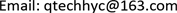1. 引言

2. 数学模型

∂ ∂ x [ ( ρ η ) e h 3 ∂ p ∂ x ] = 6 u a ∂ ∂ x ( ρ a * h ) + 6 u b ∂ ∂ x ( ρ b * h ) + 12 ∂ ∂ t ( ρ e h ) (1)

ρ a * = 2 ( ρ e − ρ ′ e η e ) , ( ρ / η ) e = 12 ( η e ρ ′ e / η ′ e − ρ ″ e )

ρ b * = 2 ρ ′ e η , ρ e = ( 1 / h ) ∫ 0 h ρ d z

ρ ′ e = ( 1 / h 2 ) ∫ 0 h ρ ∫ 0 z 1 / η d z ′ d z , ρ ″ e = ( 1 / h 3 ) ∫ 0 h ρ ∫ 0 z z ′ / η d z ′ d z

η e = h / ∫ 0 h 1 / η d z , η ′ e = h 2 / ∫ 0 h z / η d z

Reynolds方程边界条件

{ p ( x in , t ) = p ( x out , t ) = 0 p ( x , t ) ≥ 0   ( x in < x < x out ) (2)

h ( x , t ) = h 00 ( t ) + x 2 2 R + 2 π E ′ ∫ ∫ p ( x ′ , t ) ln ( x − x ′ ) d x ′ (3)

∬ p ( x , t ) d x = w 0 + k s ∗ s / L + ( m s / 3 + m t + m v ) ∗ d 2 s d t 2 * 1 L (4)

ρ = ρ 0 ( 1 + C 1 p 1 + C 2 p − C 3 ( t − t 0 ) ) (5)

η = η 0 exp { A 1 [ − 1 + ( 1 + A 2 p ) Z 0 ( A 3 t − A 4 ) − S 0 ] } (6)

c ( ρ u ∂ T ∂ x + ρ ∂ T ∂ t − w ∂ T ∂ z ) − k ∂ 2 T ∂ z 2 = − T ρ ∂ ρ ∂ T ( u ∂ p ∂ x + ∂ p ∂ t ) + τ ∂ u ∂ z (7)

{ T ( x in , z , t ) = T 0   ( u ( x i n , z , t ) ≥ 0 ) T ( x out , z , t ) = T 0   ( u ( x out , z , t ) ≤ 0 ) (8)

{ c a ρ a ( ∂ T ∂ t + u a ∂ T ∂ x ) = k a ∂ 2 T ∂ z a 2 c b ρ b ( ∂ T ∂ t + u b ∂ T ∂ x ) = k b ∂ 2 T ∂ z b 2 (9)

{ k ∂ T ∂ z | z = 0 = k a ∂ T ∂ z a | z a = 0 k ∂ T ∂ z | z = h = k b ∂ T ∂ z b | z b = 0 (10)

2. 数值方法

P = p / p H , h = h R 0 / b 2 , X = x / b , Z = z / h , Z a , b = z a , b / b

T ¯ = T / T 0 , T ¯ a , b = T ¯ a , b / T 0 , τ ¯ = τ / p H

U = u / u 0 , U 0 = u 0 η 0 / E ′ R 0 , U a , b = u a , b / u 0 , η ¯ = η / η 0

ρ ¯ = ρ / ρ 0 , W = w 0 / ( E ′ R ) , G = α E ′

3. 结果和讨论

4. 结论

a、凸轮-滚子挺杆在纯滚动运动情况下，油膜的变化主要受载荷的影响。在凸轮桃尖的两侧转角α = 119˚时，油膜厚度和中心压力均会产生较大的波动，并且在该点附近油膜厚度达到最低值。

b、当适当增加滚子的半径，油膜的厚度有了明显的提升，油膜的温升也随之变大且波动变得明显。但是由于是纯滚动，产生热量对油膜影响不大。总而言之，适当增加滚子的半径有助于凸轮-滚子挺柱的润滑。